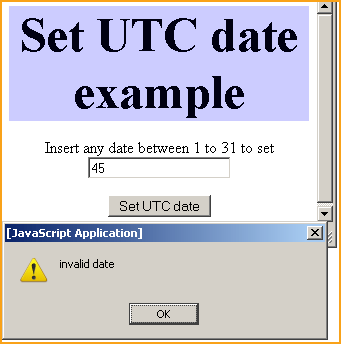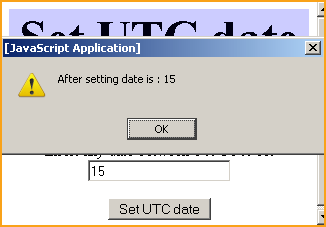# JavaScript setUTCDate method

JavaScript setUTCDate() method when applied to a date object it sets an integer value between 1 and 31 provided by the user that represents day of the date object's month.# JavaScript setUTCDate method

JavaScript setUTCDate() method when applied to a date object it sets an integer value between 1 and 31 provided by the user that represents day of the date object's month. In this part of JavaScript method tutorial we will describe you setUTCDate() method with a simple example.

Syntax :

 object_date.setUTCDate(dateValue);

Where object_date is the date object from which setUTCDate() method will be called and it will set the date of month.

In this example we have created a button "Set UTC date". This button when clicked calls the method setUTCDateFunc() which is already defined in java script. Here we have taken the current date value into the variable named "myDate". In the next line of code we have taken the date value inserted by the user into a variable UTCdateOfMonth. Now we can call the method getUTCDate() by using date object to show the current date and there after we will call setUTCDate() method to set UTC date into the myDate object variable.

 ``` function setUTCDateFunc(){ var myDate = new Date(); var UTCdateOfMonth = document.getElementById( 'UTCdateValue').value; if(UTCdateOfMonth >1 && UTCdateOfMonth < 31){ alert("Before setting date is : " +myDate.getUTCDate()); myDate.setUTCDate(UTCdateOfMonth); alert("After setting date is : " +myDate.getUTCDate()); }else{ alert("invalid date"); return false; } }```

In the above function we also have applied some validation so that user can enter only valid date to set date of month. Above lines of code is the function that we have defined in our JavaScript's <script></script> tag. Here is the full example code for setUTCDateExample.html as follows:

 ``` Set UTC date example
Set UTC date example

Insert any date between 1 to 31 to set

```

Output of example :Suppose we enter an invalid date 45 into the text box and click on the "Set UTC date" button then it alerts us with an alert message box that it is an invalid date.

If we enter 15 which is a valid date then it first shows the current date and thereafter sets the UTC date with 15.Here you can see that we have set the current UTC date with the date value 15